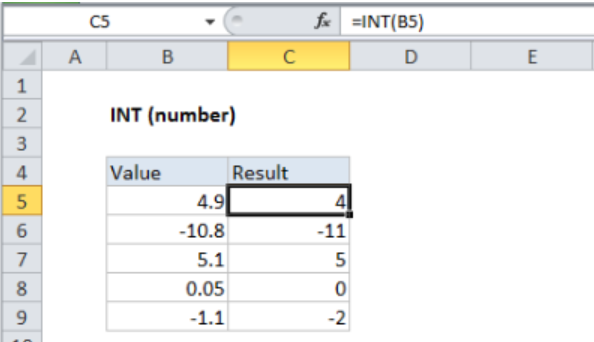Get instant live expert help with Excel or Google Sheets“My Excelchat expert helped me in less than 20 minutes, saving me what would have been 5 hours of work!”

#### Post your problem and you’ll get expert help in seconds.

Your message must be at least 40 characters
Our professional experts are available now. Your privacy is guaranteed.

# How to use Excel INT function – Excelchat

We can use Excel to calculate mathematics and round numbers down to their integers. This is made possible by the use of the Excel INT function. In this post, we shall examine how we can use the Excel integer function to get the integer part of a number.Figure 1: Excel INT function

Note that the INT function will only return the integer part of a decimal number. Also, we need to remember that the integer formula will round down a number so that those that are negative numbers will become more negative as seen in cell C9 of figure 1 above.

## The integer formula

`=INT (number)`

Where;

• Number– this is the number from which you want to get an integer.

The INT function will only round numbers down to the next lowest integer value.

## Instant Connection to an Expert through our Excelchat Service

Most of the time, the problem you will need to solve will be more complex than a simple application of a formula or function. If you want to save hours of research and frustration, try our live Excelchat service! Our Excel Experts are available 24/7 to answer any Excel question you may have. We guarantee a connection within 30 seconds and a customized solution within 20 minutes.